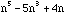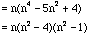Sender: Sivarajah
To: QandQ@MathCentral.uregina.ca
Date: Fri, 13 Mar 1998 18:03:00 PST

I have a question!

When each expression is evaluated for different values of n, the answers will differ. For each expression, find largest natural number that will divide the result for all natural number values of n.Name: Senthuran
Level: 10-12

Hi Senthuran

If you factor your expression you get= n(n-2)(n+2)(n-1)(n+1)

= (n-2)(n-1)n(n+1)(n+2)

Thus for each natural number n, p(n) =is the product of five consecutive natural numbers. Since, for any five consecutive natural numbers one must be a multiple of five, p(n) is divisible by five. Simularly p(n) is divisible by 4, 3 and 2. Thus p(n) is divisible by 1x2x3x4x5 = 120. When n=3, p(3) = 1x2x3x4x5 = 120 which is not divisible by any number larger than 120. Thus 120 is the largest natural number that will divide the result for all natural number values of n.

Cheers,
Harley

Go to Math Central

To return to the previous page use your browser's back button.